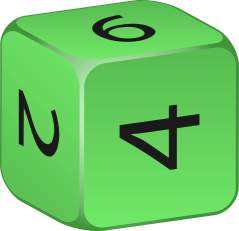# Two Dice Probability Calculator

Created by Arturo Barrantes
Reviewed by Wojciech Sas, PhD
Last updated: Jan 02, 2023

Have you ever wondered what the probability of getting a set of 2 dice with their face value of 6 pointing up while playing monopoly is? That was tons of people's dreams when they were a child. Well, the fantastic two dice probability calculator can help you understand the probability of getting that result.

The 2 dice probability calculator even helps you when you have dice of 14, 20, or more sides. So, stop waiting. It's your turn; let's roll a die: we can do it with our dice roller calculator!

## What are the probabilities of rolling 2 dice?

When rolling two dice, the number of sides/faces the die has does matter. Let's recall our Monopoly times; we had two dice with six sides. Each die has a probability of getting one face value in 6 possibilities. That makes: $1/6 = 0.167$ or $16.7\%$. If we roll two dice and do not look for any relationship between the result, we still have a $16.7\%$ probability of getting any value in each dice. We conclude probability formula is as follows:

$P= \frac{n}{N}$

where:

• $n$ represents the number of favorable events.
• $N$ includes the number of total events.
• $P$ indicates the probability of $n$ in $N$.

However, what happens when we want to get the same face value in each dice? You can use our two dice probability calculator or consider that: for getting the probability of two events simultaneously, you have to multiply each independent probability by each other. That becomes: $1/6 \times 1/6 = 0.0278$ or $2.78\%$. Consequently, the probability of rolling two dice and getting the same value (like double six in Monopoly) is less than 3%.

## How to use the two sided dice probability calculator?

To find any 2 dice probability, we follow these steps:

1. Decide the type of dice you wish to use.
2. Select the game's conditions. The options include rolling a certain value, determining whether the total of all the dice is equal to, greater than, or less than a specified target value, and a few others.
3. You might have to type which face numbers fit the winning criteria for some alternatives.
4. And that's all! The probability that results will be shown at the bottom.

If you liked the two dice probability calculator, check out our other related tools:

What were the odds to find such useful tools? Well, maybe you can find out at our odds calculator!

## FAQ

### Is there a way to get specific value in a dice?

Not exactly. We use probabilities when we refer to possible outcomes that will result randomly in the space of different possible results. But we can use the Omnicalculator tool Two dice probability calculator to determine the probability of rolling two dice above 3, and the result is 44.44%.

### How to calculate the probability of getting two 6s by rolling 2 dice?

The result is 0.0278 or 2.78%. Regarding the steps, you can try the Omnicalculator tool Two dice probability or do as follows:

1. Calculate the probability of one desired event in one dice. That is 1/6 o 0.167.
2. Power the result by the amount of dice we have. In this case, it is two. That means 0.167² or 0.0278.
Arturo Barrantes
Choose the type of dice you want to play with
Dice type
Cube (6 faces)Choose the conditions - Game rules
I want to get:
All dice with the same value
The dice value I want to get:
...which results in the probability of
... getting any value from the dice
0.1666667
People also viewed…

### First quartile

Our first quartile calculator will teach you all there is about the lower quartile.

### Meat footprint

Check out the impact meat has on the environment and your health.

### Millionaire

This millionaire calculator will help you determine how long it will take for you to reach a 7-figure saving or any financial goal you have. You can use this calculator even if you are just starting to save or even if you already have savings.

### Probability

Use the probability calculator to find the likelihood of various interactions between two distinct events.